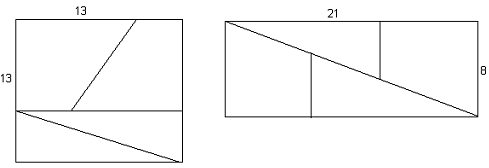### Explain the solution to the paradox using reasoning

Assignment Help Mathematics
##### Reference no: EM131995

Lewis Carroll, the author of Alice in Wonderland, liked to ask his friends to solve this puzzle:

Take a square of 13 units on one side, and cut it into sections as shown below. (It's been drawn deliberately not to scale.)

Then reposition the sections, as shown, to form a rectangle.

The area of the square is 169 sq. units.
The area of the rectangle is 168 sq. units.

How can this be??

Hint: Consecutive Fibonacci numbers are involved. (8,13,21)

Where is the missing or extra 1 sq. unit??

Investigate this puzzle and explain the solution to the paradox using mathematical reasoning. This can algebraic, geometric, or even both.Multiplication By "twos"

In many societies, years ago, the standard of numeracy was similar to that of Year 11 students today. That is, not very good. Most people could double numbers, and half them, but multiplication by larger digits was beyond most peoples' skills. Some unknown but very ingenious person used these 2 basic skills, doubling and halving, to get around the fact that while addition was known to most people, higher multiplication tables were usually not known. Here's how it worked.

Let's say we wish to multiply 19 by 47

Write two columns         19              47
Half one number,
double the other,           9              94
ignoring remainders.
4            188
2            376
1            752

We now ignore rows which have an even number in the left-hand column.
19              47
9              94
Ignore                                        4            188
Ignore                                        2            376
1            752

We now add the (non-ignored) numbers in the right -hand column .

47 + 94 + 752 = 893

So, 19

47 = 47 + 94 + 752 = 893 Which is correct !

Try this yourself for a few multiplications, and verify that it works. Use 3-digit numbers, 4 if you want. Any size. It works.

Your task is to explain, with appropriate mathematical reasoning, how this method works for any 2 positive integers.

Write the exact value of a positive number, call it H, on the board, and ask my students, one by one, to calculate the following values :

Student number 1 calculates H + 1/H

Student number 2 calculates H2 + 1/H2

Student number 3 calculates H3 + 1/H3

Student number n calculates Hn+ 1/Hn

This continues round the class. Amazingly, each student's result is an integer.

What number, H, did I write ?

Investigate the following :

There are an infinite number of possibilities for the value of H.

Explain why. The smallest value of H should be obvious.

Now we look at all the possible values of H + 1/H. The students list them in order of size and a pattern is found.

Next, we look at all the possible values of H2+ 1/H2. The students list them in order of size and again, a pattern is found.

Investigate these patterns for the values (in order of size) for values of Hn+ 1/Hn, up to at least n = 6.

Clear mathematical reasoning is obviously required.

### Write a Review